# The Use of Consulting Work to Teach Statistics to Engineering Students

Teresa Villagarcía

Journal of Statistics Education v.6, n.2 (1998)

Copyright (c) 1998 by Teresa Villagarcía, all rights reserved. This text may be freely shared among individuals, but it may not be republished in any medium without express written consent from the author and advance notification of the editor.

Key Words: Convolution; Monte Carlo simulation; Power generating systems; Reliability.

## Abstract

This article presents the use of an interesting consulting problem as a practical exercise for a basic course in statistics for engineering students. The consulting problem considered is the estimation of the reliability of the Spanish power generating system. We have used this problem to illustrate the distribution of sums of random variables, the central limit theorem and its limitations, and other issues. We have also designed a practical exercise to show the students the use of Monte Carlo simulation to solve part of the statistical problem.

# 1. Introduction

1 The use of real problems has been widely recommended to teach statistics to students, especially to non-specialists (Hogg 1985). Often, however, teachers do not have contact with real problems connected to the students' fields, so "real problems" are in fact "real book problems," and the freshness is lost. There is a real possibility of finding suitable problems in consulting work. These problems are not usually developed at a very high mathematical level and thus are entirely adequate for teaching purposes.

2 In this paper we consider one of our consulting projects from 1993, and its usefulness in a statistics course for engineering students. This project provides an illustration of concepts related to the central limit theorem and simulation techniques. The consulting problem considered is the estimation of the reliability of the Spanish power generating system.

# 2. The Problem

3 The function of a power generating system (PGS) is basically to supply customers with electrical energy as economically as possible, and with a high degree of reliability and quality. Therefore generating systems are designed and operated to keep the risk of not being able to meet the load demand below a predetermined level.

4 The reliability assessment of a power generating system can be divided into two main aspects: system capability, which is related to the existence of sufficient facilities to satisfy customer load demand, and system security.

5 This study focuses on evaluating the system's capability to meet demand. A modern power system is complex and very large. The power can be generated from fuels (fossil or nuclear) or by hydroelectric stations. We will focus only on fuel plants. Therefore the PGS will be composed of n fuel power generating stations. Each of the n stations has an installed capacity Ci megawatts (MW), and the system installed power supply (CT ) is the sum of the installed capacities of all the stations.

6 It is well known that electricity cannot be stored unless an extremely high cost is assumed. Only batteries and reservoirs can store electric energy. Therefore a PGS is designed to supply instantaneously the power demanded by consumers.

7 Failures in the system occur when demand exceeds supply. Demand can exceed supply for two main reasons. First, if there is a very high demand peak that exceeds the installed capacity of the system, the system cannot supply the load peak. Second, if some power stations are out of service because of failures or periodic maintenance, a high demand that does not exceed the installed capacity of the system can exceed the available capacity at that moment. A system failure of either type is referred to as a loss of load.

8 The reliability of a power generating system can be defined in many ways (Billinton and Allan 1984). Some widely used reliability indices are the Loss of Load Probability (LOLP), which is the probability that the system cannot supply the load peak during a given interval of time, and the Loss of Load Expectation (LOLE), which is the expected time with a loss of load.

9 Let S be a random variable representing the available power supply, and let L be the daily load peak. If the peak load is regarded as known, then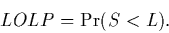(1)

Usually the system capacity is designed to have a very small LOLP.

10 To estimate the LOLP, the first step is the estimation of S, and this is the problem that we have used for teaching purposes.

11 We assume that any station has two possible working states: operational or down. The probability of the station being down is known as the Forced Outage Rate (FOR). The FOR can be estimated from the station's history. We have estimated the FOR for all the fuel stations, using records from the electric companies that contain the exact minute of the beginning and end of every failure in the last five years. There are also theoretical FORs which are obtained from the plant's designers on the basis of technical characteristics of the plants. The estimated FORs are in general smaller than the theoretical ones, so that the reliability of the system is underestimated for security purposes.

12 Let Xi be a random variable representing the available power that can be supplied by the ith plant.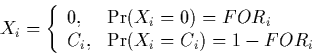where Ci is the capacity and FORi the Forced Outage Rate of plant i.

13 Now the available supply S,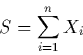is the sum of n independent random variables. In the particular case of our variables, if Gk(x) is the distribution function of, then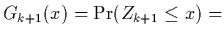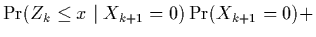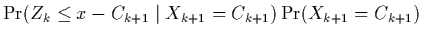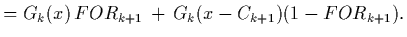(2)

14 In Spain there are n = 80 power generating stations, and traditionally it has been considered that this involves 280 possible values for S, and the sum of random variables (that is, the convolution) becomes almost impossible to compute. Approximate procedures will not be very effective in estimating the far tail probabilities that are required in LOLP calculations.

15 The existence of several large units (nuclear stations) and otherwise mostly small units in a typical PGS mix violates the spirit of the Lindeberg condition (Feller 1971, Cramer 1946) of the central limit theorem. Furthermore, in a practical problem with only a finite number of random variables, the normal distribution provides a good approximation when the value of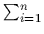FORi(1 - FORi) is large. So, since the value of each term FORi(1 - FORi) is a maximum when FORi = 0.5, the approximation will be best when n is large and the values of FOR1, FOR2, ... , FORn are close to 0.5 (De Groot 1986). This is obviously not the case, because a typical theoretical FOR value is 0.05. The assumption of normality may not necessarily cause problems if one is computing probabilities in the central part of the distribution, but probabilities may be estimated inaccurately in the tail.

16 Many approximations have been suggested to deal with this problem. Edgeworth expansions have been used, but they do not work well in the tails of distributions. Mazumdar and Gaver (1984) used saddlepoint approximations with apparently much better results. We have coded a very efficient and fast algorithm in MATLAB that computes the exact distribution function of S. Using MATLAB on a Pentium 75, the convolution of the 80 stations takes 2.33 seconds. The algorithm, based on equation (2), is included in the Appendix. However, none of these approximations is appropriate for a basic statistics course for engineering students.

# 3. The Students' Work

17 To make the problem appropriate for students, we have introduced the concept of the sum of random variables by obtaining the probability mass function of X1 + X2 and X1 + X2 + X3. Students quickly realize that there are a lot of supply values when more and more power stations are added.

18 We also talk about the central limit theorem and explain the conditions due to Lindeberg. The conditions are relevant in this context because we are estimating a very small probability in the right tail of the distribution.

19 With very little guidance, students generate 80 random numbers rj, j = 1, ... , 80, rj ~ U(0,1), and define sj in the following way: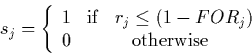Therefore sj represents the state of the jth plant in the current simulation. If sj = 1, the plant is up; otherwise, the plant is down. The probability that the jth station is working properly is 1 - FORj.

20 Let(3)

represent the ith simulation of the supply. Repeating the process 5000 times using MATLAB produced the histograms shown in Figures 1 and 2.

21 Figure 1 shows the simulated power supply in MW, using the theoretical FOR for each of the 80 power plants. It also shows the exact probability mass function, calculated by the convolution of the stations. The problem of discontinuities in the tails of the distribution can be easily appreciated in the figure, and was explained in the classroom. This is a simple exercise: first calculate Pr(S = CT ), then note that Pr(S = CT - k) = 0 for k C1, C2, ... , Cn).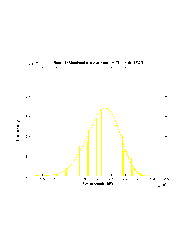Figure 1 (7.3K gif)

Figure 1. Simulated and Exact Power Supply (Theoretical FOR).

22 Figure 2 presents the simulated power supply using the estimated FORs instead of the theoretical ones and, for comparison purposes, the exact probability mass function presented in Figure 1. As can be seen from the figures, the simulated supply using the estimated FORs is shifted to the right, so that the system capacity is larger than when the theoretical FORs are used. It is suggested that the students evaluate the differences in the two solutions: the central limit theorem and the exact probability mass function.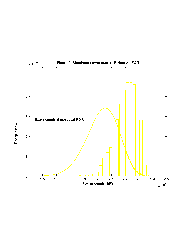Figure 2 (8.3K gif)

Figure 2. Simulated Power Supply (Estimated FOR).

23 We have also estimated the effect of an extra station on the available supply to show the students how this method can be used for design purposes. Figure 3 shows the effect of an extra station of 1000 MW and FOR = 0.05, using the theoretical FOR and the exact probability mass function of Figure 1.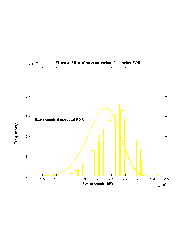Figure 3 (8.0K gif)

Figure 3. Effect of an Extra Station (Theoretical FOR).

# 4. Discussion

24 The use of real problems in teaching statistics has been recommended by many authors (Godfrey 1986, Hogg et al. 1985). Our experience with ten practical classes in which we have tried to study real data and problems has been very positive. We have used this work to illustrate many concepts: the sum of random variables, the central limit theorem, simulation techniques, and discrete convolution. We have also used other consulting work, mainly in quality control and the evaluation of customer expectations, to illustrate regression and quality control.

25 Surveys carried out by the university to evaluate the quality of lecturers and programs have shown that statistics ranked first in practical sessions (before physics and chemistry), and that the computer-lab sessions have very much helped the students to understand the theory. We have noticed that the motivation of students who have solved real engineering problems, such as the one described, has increased greatly in the subsequent theoretical classes.

## Acknowledgments

I am grateful to the editor and three referees for their comments that resulted in a much improved version of the work. The research reported in this paper was partially supported by Spanish MEC Grant PB-96-011.

# Appendix

Below is a MATLAB program that computes the exact distribution function of S. The program performs the following steps.

Step 1
Find the distribution function of S when we have one station in the system. This function is stored in g1. g1 is a vector of length C1 and value FOR1. Note that g1(i) = G1(i), i = 1, ... , C1.

Step 2
Add the next station. (The first time, i = 2.) Based on equation (2), displace the values of g1 by adding Ci zeroes at the beginning of g1. The size of g1 is now C1 + ... + Ci. Call this vector g1dis. Then multiply g1dis by 1 - FORi. This is the second term of the right hand side of the last line of equation (2). The first term is obtained by adding to g1 (the distribution function of the system when there are i - 1 stations), Ci ones at the end of g1, and multiplying the resulting vector by FORi. The sum of both terms is called g2; this is the distribution function from 1 to C1 + ... + Ci of the system when there are i stations.

Step 3
Repeat Step 2 until all the stations have been added (i = n). With this method, we obtain all the values of Gn(x), x <Cj. The values are the components of vector g2 at the end of the process, so that g2(i) = Gn(i). The size of g2 at the end of the process isCj.

n=length(pow);
g1=[forest(1)*ones(size(1:pow(1)))];
for i=2:n;
g1dis=[zeros(size(1:pow(i))) g1];
g1=[g1 ones(size(1:pow(i)))];
g2=forest(i)*g1+(1-forest(i))*g1dis;
g1=g2;
end;


pow is a 1 x 80 vector containing the stations' capacities in megawatts.

forest is a 1 x 80 vector containing the estimated FORs.

g1 is a 1 x CT vector containing the theoretical distribution function.

# References

Billinton, R., and Allan, R. N. (1984), Reliability Evaluation of Power Systems, London: Pitman Advanced Publications.

Cramer, H. (1946), Mathematical Methods of Statistics, Princeton, NJ: Princeton University Press.

De Groot, M. H. (1986), Probability and Statistics (2nd ed.), Reading, MA: Addison-Wesley.

Feller, W. (1971), An Introduction to Probability Theory and Its Applications (Vol. II, 2nd ed.), New York: John Wiley.

Godfrey, B. (1986), "Future Directions in Statistics," Center for Quality and Productivity Improvement, Report No. 10, University of Wisconsin, Madison.

Hogg, R. V. et al. (1985), "Statistical Education for Engineers: An Initial Task Force Report, The American Statistician, 39, 168-175.

Mazumdar, M., and Gaver, D. P. (1984), "On the Computations of Power-Generating System Reliability Indexes," Technometrics, 26, 173-185.

Teresa Villagarcía